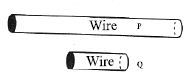# Explain Dependence of Resistance

We know, when temperature and other physical conditions (e.g. – length, cross-section, area) remain the same, the resistance of a conductor is a constant. The resistance of a conductor depends on four factors.

1. Length of the conductor.
2. A cross-sectional area of the conductor.
3. Materials of the conductor and.
4. Temperature of the conductor.

We know, if the temperature remains constant, the resistance of a conductor depends only on its length, area of cross-section and the material of the conductor. This dependence of resistance is expressed by two laws.

Figure shows two conducting wires P and Q with the same cross-sectional area and made of the same material. The length of wire P is longer than that of Q. Its resistance is greater as it is longer.Law of length: The resistance of a conductor is directly proportional to its length when the cross sectional area, material and temperature of the conductor remain the same. If the length of the conductor is L, area of cross section is A, and its resistance is lt, then according to this law,

R ∞ L , when temperature, material and A is constant .

Law of cross section: The resistance of a conductor is inversely proportional to its cross sectional area when the length, material and temperature of the conductor remain the same. That is, R ∞ 1/A, when temperature, material and L is constant.